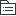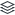# 如何高效学习

207
|
17

## 相关模板推荐

•## —— 作品大纲

•策略篇
• 整体性学习
• 知识结构
• 模型
• 毋须非常准确
• 便于理解
• 纠错、升级
• 高速公路
• 信息跨界
• 交叉整合
• 成熟结构
• 感知结构
• 图像、声音
• 生活经验
• 关系结构
• 卤化物&惰性气体
• 基础数学
• eg。1+1>2
• 学习的顺序
• 获取
• 简化
• 容量
• 速度
• 理解
• 拓展
• 深度
• 探究知识的背景
• 横向
• 知识周围建立联系
• 纵向
• 比喻法
• 内在化
• 发现类似结构
• 纠错
• 存在未必合理
• 思路
• 加栗子
• 删减联系
• 方法
• 阅读相反观点
• 现实检验
• 应用
• 抛开书本、融入生活
• 自领域
• 跨领域
• 测试
• 信息结构
• 五类信息
• 随意信息
• 事实、日期、定义、规则
• 联想法
• 挂钩法
• 信息压缩
• 观点信息
• 存在争论的信息
• 图表法
• 过程信息
• 行动、操作
• 内在化
• 比喻法
• 图表法
• 模型纠错
• 具体信息
• 现实感官、接触
• 内在化
• 抽象信息
• 缺少感官
• eg。数学、心理学
• 内在化
• 比喻法
• 模型纠错
• 使用
• 学习新东西
• 信息种类
• 了解困难
• 选取技术
• 差异化
• 弱结构
• 强结构
• 学习目标
• 获得知识
• 三思而学
• 动机
• 知识，用而获得新的意义
•技术篇
• 获取知识
• 笔记流
• 一次学会
• 基本策略
• 主要观点
• 关键词
• 箭头
• 重点
• 更好理解
• 好看/标准
• 混合型
• 记录
• 拓展
• 联系、图表
• 课下
• 课上，常规笔记
• 课下，笔记流
• 评注
• 重点
• 联系
• 快速阅读
• 指读法
• 视线聚焦
• 练习阅读法
• 三分钟阅读
• 默写
• 详读
• 对比
• 积极阅读法
• 主要观点
• 怎样记忆
• 拓展、应用
• eg.心锚、条件反射
• 联系观点
• 比喻
• 注意第一念头
• 优化
• 图表
• 流程图
• 概念图
• 图像
• 内在化
• 明确概念
• 建立图像
• 加上感官
• 重复、优化
• eg.NLP12条假设
• 随意信息
• 联想法
• 顺序
• 抽象
• 夸张的联想
• 挂钩法
• 数字桩
• 图像记忆
• 信息压缩
• eg.SMART原则
• 知识延伸
• 实际使用
• 模型纠错
• 分清错误类型
• 散弹枪策略
• 不少做
• 不多做
• eg。软件测试
• 延伸练习时间
• 费曼技巧
• 找出概念
• 站在老师角度
• 有疑惑→返回
• 简单化/比喻
• eg.相对论
• 定项学习
• 从小目标开始
• 记录学习进度
• 创造有意义的目标
•超越篇
• 高效率
• 能量管理
• 能量储备
• 循环式日程表
• 不要“学习”
• 具体化
• 杜绝空泛
• 绝不拖延
• 清单
• 排序
• 批处理
• 类似的事情
• 有组织
• 固定位置
• 记录
• 坚持日历
• 自我教育
• 学习目标
• 写下目标
• 客观化